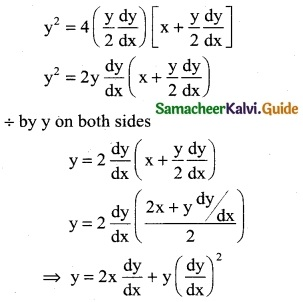Tamilnadu State Board New Syllabus Samacheer Kalvi 12th Business Maths Guide Pdf Chapter 4 Differential Equations Ex 4.1 Text Book Back Questions and Answers, Notes.

## Tamilnadu Samacheer Kalvi 12th Business Maths Solutions Chapter 4 Differential Equations Ex 4.1

Question 1.
Find the order and degree of the following differential equations.
(i) $$\frac { dy }{dx}$$ + 2y = x³
Solution:
Highest order derivative is $$\frac { dy }{dx}$$
∴ order = 1
Power of the highest order derivative $$\frac { dy }{dx}$$ is 1
∴ degree = 1(ii) $$\frac { d^3y }{dx^3}$$ + 3($$\frac { dy }{dx}$$)³+ 2$$\frac { dy }{dx}$$ = 0
Solution:
Highest order derivative is $$\frac { d^3y }{dx^3}$$
∴ order = 3
Power of the highest order derivative $$\frac { d^3y }{dx^3}$$ is 1
∴ degree = 1

(iii) $$\frac { d^2y }{dx^2}$$ = $$\sqrt{y – \frac { dy }{dx}}$$
Solution:
[ $$\frac { d^2y }{dx^2}$$ ]² = y – $$\frac { dy }{dx}$$
Highest order derivative is $$\frac { d^2y }{dx^2}$$
∴ order = 2
Power of the highest order derivative $$\frac { d^2y }{dx^2}$$ is 2
∴ degree = 2(iv) $$\frac { d^3y }{dx^3}$$ = 0
Solution:
Highest order derivative is $$\frac { d^3y }{dx^3}$$
∴ order = 3
Power of the highest order derivative $$\frac { d^3y }{dx^3}$$ is 1
∴ degree = 1

(v) $$\frac { d^3y }{dx^3}$$ + y + [ $$\frac { dy }{dx}$$ – $$\frac { d^3y }{dx^3}$$ ]3/2 = 0
Solution:Highest order derivative is $$\frac { d^3y }{dx^3}$$
∴ order = 3
Power of the highest order derivative $$\frac { d^3y }{dx^3}$$ is 3
∴ degree = 3

(vi) (2 – y”)2 = y”² + 2y’
Solution:
(2)² – 2(2) (y”) + (y”)² = (y”)² + 2y’
4 – 4y” = 2y’
Highest order derivative is y”
∴ order = 2
Power of the highest order derivative y” is 2
∴ degree = 2(vii) ($$\frac { dy }{dx}$$)³ + y = x – $$\frac { dx }{dy}$$
Solution: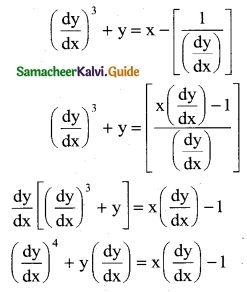Highest order derivative is $$\frac { dy }{dx}$$
∴ order = 1
Power of the highest order derivative $$\frac { dy }{dx}$$ is 4
∴ degree = 4

Question 2.
Find the differential equation of the following
(i) y = cx + c – c³
(ii) y = c (x – c)²
(iii) xy = c²
(iv) x² + y² = a²
Solution:
(i) y = cx + c – c3 ……. (1)
Here c is a constant which has to be eliminated
Differentiating w.r.t x, $$\frac{d y}{d x}$$ = c …… (2)
Using (2) in (1) we get,
$$y=\left(\frac{d y}{d x}\right) x+\frac{d y}{d x}-\left(\frac{d y}{d x}\right)^{3}$$ which is the required differential equation.(ii) y = c (x – c)² ……… (1)
y = c (x² – 2cx + c²)
y = cx² – 2c²x + c³
Differentiating w.r. to x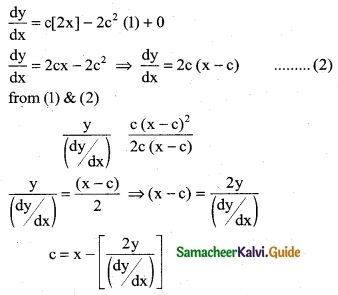Substituting this value of c and (x – c) in (1), we get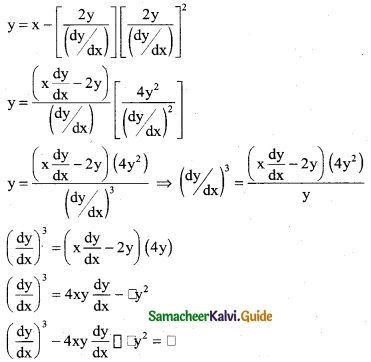(iii) xy = c²
Differentiating w.r. to x
x($$\frac { dy }{dx}$$) + y(1) = 0
∴ x($$\frac { dy }{dx}$$) + y = 0

(iv) x² + y² = a²
Differentiating w.r. to xQuestion 3.
Form the differential equation by eliminating α and ß from (x – α)² + (y – α)² = γ²
Solution:
(x – α)² + (y – α)² = γ² ……… (1)
where α and ß are parameters.
Since equation (1) contains two orbitary constants,
We differentiate it two times w.r.t. x
Differentiating (1) w.r.t. x, we getSubstituting the value of (x – α) and (y – ß) in (5) we get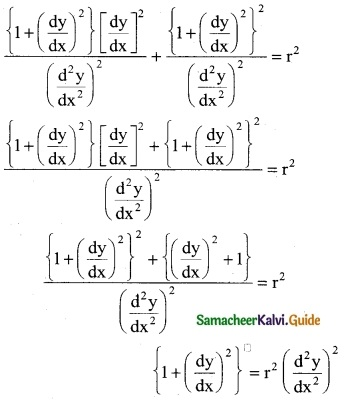This is the required differential equation.Question 4.
Find the differential equation of the family of all straight lines passing through the origin.
Solution:
The general equation for a family of lines passing through the origin is
y = mx ……. (1)
Differentiating w.r.t x,
$$\frac{d y}{d x}$$ = m ……. (2)
Using (2) in (1)
y = ($$\frac{d y}{d x}$$) x is the required differential equationQuestion 5.
Form the differential equation that represents all parabolas each of which has a latus rectum 4a and whose axes are parallel to the x-axis.
Solution:
The equation of the family of the parabola is
(y – k)² = 4a (x – h) ……. (1)
where h and k are arbitrary constants,
[we have to differentiate the equation twice to eliminate h and k]
Differentiating equation (1) w.r.t. xQuestion 6.
Find the differential equation of all circles passing through the origin and having their centers on the y axis, x² + (y – k)² = r²
Solution:
Equation of circle whose centre is (h, k)
(x – h)² + (y – k)² = r²
since the centre is on the y-axis (ie) (0, k) be the centre
(x – 0)² + (y – k)² = r²
x² + (y – k)² = r² ………. (1)
since the circle passing the origin (0, 0)
Eqn (1) becomes
0 + (0 – k)² = r²
k² = r² ⇒ r = k
Eqn (1) ⇒ x² + (y – k)² = k²
x² + y² – 2yk + k² = k²
x² + y² – 2yk = 0
x² + y² = 2yk ……….. (2)
Differentiating w.r.t. xQuestion 7.
Find the differential equation of the family of parabola with foci at the origin and axis along the x axis, y² = 4a (x + a)
Solution:
Equation of parabola with foci at the origin and axis along the x-axis is
y² = 4a(x + a) ……… (1)
Differentiate w.r.t. x
2y $$\frac { dy }{dx}$$ = 4a (1 + 0)
2y = $$\frac { dy }{dx}$$ = 4a ⇒ a = $$\frac { y }{2}$$, $$\frac { dy }{dx}$$
Substitute the value of a = $$\frac { y }{2}$$ $$\frac { dy }{dx}$$ in equ (1)